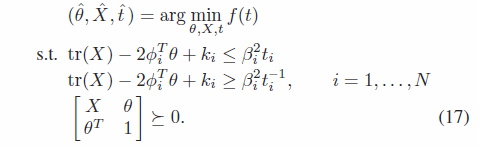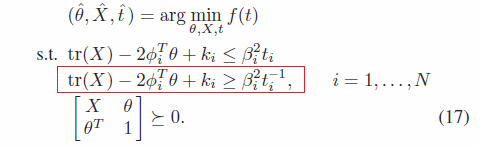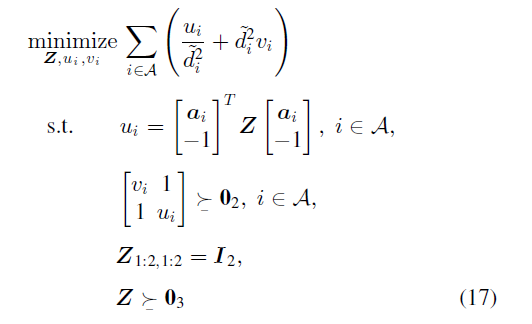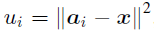# Func. Optimization for target localization

Hello everybody, I am reproducing this paper http://www.bigdatalab.ac.cn/~wouyang/pubs/Received%20Signal%20Strength-Based%20Wireless%20Localization%20via%20Semidefinite%20Programming%20Noncooperative%20and%20Cooperative%20Schemes.pdf.
To be exact, I am implementing the eq. 17..
The first error is “Error using sym>tomupad (line 1251)
Unable to convert ‘cvx’ to ‘system’.” To avoid this error and see if the rest of the code works I set up X=eye(2) and I got another error: "Error using reshape
To RESHAPE the number of elements must not change.

Error in cvx/cat (line 88)
yb = reshape( yb, nz, psz );

Error in cvx/vertcat (line 6)
y = cat( 1, varargin{:} );".

Here, part of the code:

cvx_begin
variables theta(2) t(N); % theta= [x y] target loc. % N=number of anchors
X= thetatheta’; % X -> auxiliary variable
% X= eye(2);
minimize(norm(t, inf))
subject to
for i =1:N
trace(X) - 2
phi(i,:)*theta +Ki(i) <= Bi_sqr(i)t(i);
[ trace(X) - 2
phi(i,:)*theta +Ki(i), sqrt(Bi_sqr(i));
sqrt(Bi_sqr(i)), t(i)] >= 0;
[ X, theta;
theta’, 1 ]>= 0;
end

cvx_end

I am new with this tool, my apologies if my questions are so basic. Any help you can provide will be greatly appreciated.

You should never be forming `X= theta*theta’.` That is non-convex. Instead, this formulation relaxes that via Schur Complement into the LMI, `[X, theta;theta’, 1 ] >= 0`. Note that you need to use `cvx_begin sdp` if you want to enter it this way, otherwise use `[X, theta;theta’, 1 ] == semidefinite(3)`

So use `variable X(2,2) symmetric` .and get rid of `X= theta*theta’`;

I have not checked to see whether you have any other errors.

1 Like

I made changes in the code following your suggestion. Here is the new code:

cvx_begin
variables theta(2) t(N); % theta= [x y] target loc. % N=number of anchors
variable X(2,2) symmetric

``````minimize(norm(t, inf))

subject to
for i =1:N

trace(X) - 2*phi(i,:)*theta +Ki(i) <= Bi_sqr(i)*t(i);
[ trace(X) - 2*phi(i,:)*theta +Ki(i), Bi(i) ;
Bi(i), t(i)] >= 0;
``````

% trace(X) - 2*phi(i,theta +Ki(i) >= Bi_sqr(i)(1/t(i));

``````    end
[ X, theta ; theta', 1 ]>= 0;
``````

cvx_end

The first error does not appear anymore, however, the second one is still present. This error is related to the second constraint(red box):Error using reshape
To RESHAPE the number of elements must not change.

Error in cvx/cat (line 88)
yb = reshape( yb, nz, psz );

Error in cvx/vertcat (line 6)
y = cat( 1, varargin{:} );

This error is for this line of code which is the LMI for the second constraint:

[ trace(X) - 2*phi(i,:)*theta +Ki(i), Bi(i) ;
Bi(i) , t(i) ] >= 0;

I also try to use its original form ( trace(X) - 2*phi(i,: )theta +Ki(i) >= Bi_sqr(i)(1/t(i)); ) then it appears the following error:

Error using ./ (line 42)
Disciplined convex programming error:
Invalid operation: {1} / {real affine}

Any suggestion to avoid this error will be kindly appreciated.
thank you very much for your time

For the 2nd form, you can use inv_pos. If you do so, CVX accomplishes behind the scenes what the LMI version of that is doing.

Your LMI has a reshape error because spaces before and after - and + are causing MATLAB to create additional elements, as would occur with comma, So remove all the spaces within an element of the matrix.

Also, given the `>= 0` syntax you’ve used to specify LMIs (semidefinite constraints), you need to use `cvx_begin sdp` That was the point of the 3rd sentence of my previous post, but I somehow forgot to include `sdp` prior to editing it just now.

1 Like

Dear Mark,

Thank you very much for your time, I really appreciate it. Thanks to your advice, I could solve my problem.
Do you know a web or book where I can check CVX optimization codes, by any chance? I would like to practice more.

Once again, thank you very much.

You can look at http://cvxr.com/cvx/examples/ for CVX examples. I think there are some optimization books which have some CVX examples, but I have not read any of them,

If you read http://stanford.edu/~boyd/cvxbook/ and do some of the exercises, you can greatly improve your ability to formulate and transform convex optimization problems suitable for CVX. And you will probably get some insight to help you understand what CVX is doing under the hood (you can look at CVX source code for functions such as inv_pos to see what it does).

Re-read the CVX USer’s Guide, now that you’ve actually used CVX a bit.

You can also read old CVX forum postings - many will be boring and uninsightful, but several of them might prove interesting and informative. Unfortunately, the thread titles are often not very informative as to the contents.

1 Like

Once again, thanks a lot!

The MOSEK modeling cookbook https://docs.mosek.com/modeling-cookbook/index.html may also help you understand how to formulate and transform conic optimization problems in a way which will help you use CVX effectively, even though it has no CVX examples. It should help you understand papers such as the one you linked, and how to implement models described therein in CVX. http://stanford.edu/~boyd/cvxbook/ (previously linked) can do that as well.

1 Like

Dear Mark,
I am reproducing a paper entitled “Cooperative RSS-Based Localization in Wireless Sensor Networks Using Relative Error Estimation and Semidefinite Programming”. It seems that I can not upload the file.
I am implementing the following equation:In the paperwhere ‘a_i’ and ‘x’ are bidimensional vectors, and ‘v_i=1/u_i’.
Please find below my code for this equation:

cvx_begin sdp quiet
variables u_i(len_ANs) v_i(len_ANs) % ‘len_ANs’ is the length of the set ‘A’
variable Z(3,3) symmetric

``````        cost=0;
for i=1:len_ANs
cost=cost+(u_i(i)/(d_tilt(i)^2)+(d_tilt(i)^2)*v_i(i));
end

minimize(cost)

subject to
for i =1:len_ANs
u_i(i)==[a_i(i,:) -1]*Z*[a_i(i,:)';-1];
[v_i(i) 1; 1 u_i(i)]>=zeros(2,2);
end
Z(1:2,1:2)==eye(2);
Z>=zeros(3,3);
``````

cvx_end

In this occasion, I don’t have any errorhowever the results that I have obtained are quite different from what is shown in the paper. I have contacted the authors for this matter but unfortunately, I couldn’t get any response. I know that this difference can be for some simulations setups but I would like to discard any problem within the code. Please, could you let me know if you find any inconsistency with the code and the equation?
Additionally, I wonder if a problem is formulated using a second-order cone programming the way how I call the CVX package affects the result, for instance, what about if I use just " cvx_begin" instead of " cvx_begin sdp"?
Thank you very much.

I don’t see anything obviously wrong from a quick perusal, except that I have no idea how u_i = ||a_i - x||^2 is supposed to come into play.

You have not provided a reproducible problem, because you didn’t provide the input data. And you haven;t shown the output, and how it compares to the paper. Furthermore, you have used `quiet`, so we don’t know what the solver and CVX reported in terms of whether the problem was solved.

Dear Mark,
thank you very much for your kind reply. Well, the main idea is to retrieve de estimation of ‘x’ (which is related to u_i by) from ‘Z’ after solving (17). The input for (17) is ‘d_tilt’ and the output the estimation of ‘x’. Please find below a complete version of the code for one Monte Carlo simulation:
%%%%%%%%%%%%%%%%%%%%%%%%%%%%%%%%%%%%%%%%%%%%%%%%

``````%Parameter setup
a_i= [0 0; 100 0; 100 100; 0 100; 50 50];
len_ANs= length(a_i);

y=4;
do=1;
P0=-40;
mean_ni=0;
sigma_ni=sqrt(6) ;

TN=[10 30] % 'x'

% Data generation
for i= 1: len_ANs
n_i(i)= normrnd(mean_ni,sigma_ni); % noise generation
P_i(i)= P0 - 10*y*log10(norm(TN-a_i(i,:),2))+ n_i(i); % RSS generation
d_tilt(i)= 10^((P0-P_i(i))/(10*y)); % input to eq. (17)
end

%%%%%%%%%%%%%%%%%%%%%%%%%%%%%%%%%%%%%%%%%%%%%%%%
cvx_begin sdp %quiet
variables u_i(len_ANs)  v_i(len_ANs)
variable Z(3,3) symmetric

cost=0;
for i=1:len_ANs
cost=cost+(u_i(i)/(d_tilt(i)^2)+(d_tilt(i)^2)*v_i(i));
end

minimize(cost)

subject to
for i =1:len_ANs
u_i(i)==[a_i(i,:) -1]*Z*[a_i(i,:)';-1];
[v_i(i) 1; 1 u_i(i)]>=zeros(2,2);
end
Z(1:2,1:2)==eye(2);
Z>=zeros(3,3);
cvx_end
ui_SDP_wang=Z(1:2,3)'; % estimation of 'x'
%%%%%%%%%%%%%%%%%%%%%%%%%%%%%%%%%%%%%%%%%%%%%%%%
RMSE_SDP_Wang=sqrt(norm(TN-ui_SDP_wang,2).^2) % Paper output
``````

I have noticed that most of the time the status of the solver is Inaccurate/Solved. May I know why is it happening and how to solve it? Below what CVX reports:

## Calling SDPT3 4.0: 21 variables, 8 equality constraints For improved efficiency, SDPT3 is solving the dual problem.

num. of constraints = 8
dim. of sdp var = 3, num. of sdp blk = 1
dim. of socp var = 15, num. of socp blk = 5

SDPT3: Infeasible path-following algorithms

## number of iterations = 14 primal objective value = -2.42180423e+00 dual objective value = -2.30649890e+00 gap := trace(XZ) = 3.93e-06 relative gap = 6.85e-07 actual relative gap = -2.01e-02 rel. primal infeas (scaled problem) = 2.33e-08 rel. dual " " " = 6.33e-10 rel. primal infeas (unscaled problem) = 0.00e+00 rel. dual " " " = 0.00e+00 norm(X), norm(y), norm(Z) = 1.1e+04, 1.1e+03, 2.3e+04 norm(A), norm(b), norm© = 6.0e+02, 1.6e+04, 3.5e+04 Total CPU time (secs) = 0.12 CPU time per iteration = 0.01 termination code = -7 DIMACS: 2.9e-08 0.0e+00 1.1e-09 0.0e+00 -2.0e-02 6.9e-07

Status: Inaccurate/Solved
Optimal value (cvx_optval): +9.89173

Using the input you have provided, the problem is solved to reported oiptimality by all three of SDPT3, SeDuMi, and Mosek, and all within agreement with each other within tolerance. The optimal u_i values go up to ~ 1e4, which is a little large, but doesn’t seem to present a problem (although perhaps it does in post-processing?)

I haven’t tried to figure out what you are doing with your post-CVX calculations, because that is not part of your CVX optimization problem, But perhaps you are doing something different from the paper there.

Dear Mark,
As I mentioned before the status of the solver, most of the time is “Status: Inaccurate/Solved”. To consider a proper solution the status shouldn’t be just “Status: Solved”?

You provided one problem instance (one set of input data), and I wrote what happened when I ran the problem. If Status is Inaccurate/Solved, the solver was not able to achieve the requested accuracy. That cold be because of a numerically challenging problem, or just because the solver isn’t that robust. It may happen that SDPT3 gets Inaccurate/Solved, but Mosek, which is more numerically robust can acheve solved. BTW, don’t assume that all the runs made by the paper’s authors were necessarily “clean”. For al we know, you may be the 2nd person in the world (and me, the 3rd) to ever attempt the method in the paper.

Dear Mark,